CBSE Class 12 Chemistry Practice Paper 2023: The Class 12th chemistry board exam is to be held on 28th February 2023. It is important to solve the practice papers during the last-minute preparation for the exams. The Central Board of Secondary Education (CBSE) has released the “Additional Practice Questions” for the Class 12 Chemistry exam 2023 to help students check their preparation level and get an idea of difficult topics. The sample papers provide the blueprint and mars distribution of exams and consist of mainly generic questions. Plus, they are released several months before the exam. The 2023 CBSE class 12 chemistry practice paper, on the other hand, consists of important questions which help in some much-needed revision in the last few days of the exam. These practice papers are intended to assist students to gauge their level of preparedness as well as gaining a better idea of the types of questions that will be asked in the final exams. You can read and download the CBSE class 12 chemistry practice paper 2023 PDF along with the marking scheme here.

Related: CBSE Chemistry Class 12 Syllabus 2023

## CBSE Class 12 Chemistry Practice Paper 2023

There are 35 questions in the CBSE Class 12 Chemistry Paper totaling 70 marks. The duration of the exam will be 3 hours and internal choice between questions will be provided.

Section A consists of 18 multiple-choice questions carrying 1 mark each.

Section B consists of 7 very short answer questions carrying 2 marks each.

Section C consists of 5 short answer questions carrying 3 marks each.

Section D consists of 2 long answer questions carrying 4 marks each.

Section E consists of 3 long answer questions carrying 5 marks each.

### Chemistry (043) Practice Questions 2023

SECTION A

The following questions are multiple-choice questions with one correct answer. Each

question carries 1 mark. There is no internal choice in this section.

Q.1 De-icing is the process of removing snow, ice or frost from a surface. In extremely cold regions, car windows get covered by ice reducing visibility. The image below shows

the de-icing of the window of a car during extreme cold using a fluid.

Which of the following compounds could be present in the de-icing fluid used above?

1. formaldehyde
2. phenol
3. propan-2-ol
4. acetic acid

Q.2 Which of the following reaction mechanism is not involved in the given reaction Sequence?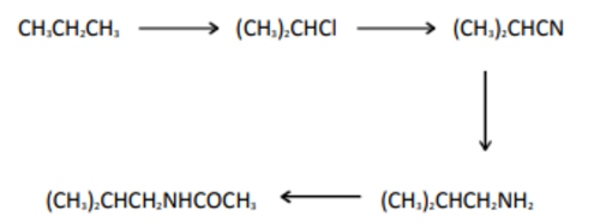2. nucleophilic substitution
3. elimination

Q.3 The graph below shows the observed standard electrode potential of some transition elements.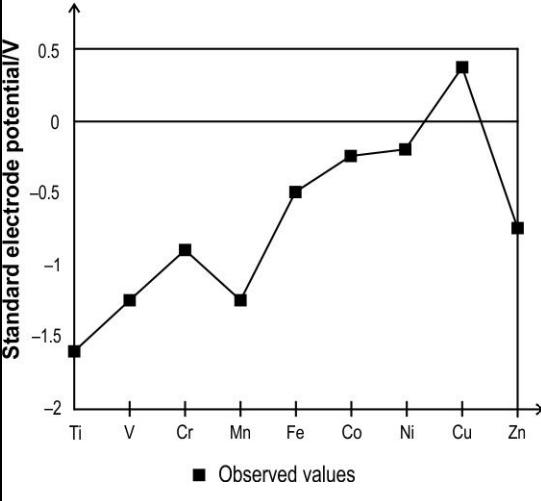Which of the following reactions can be predicted based on the graph above?

1. Cu + 2 H2SO4 –> CuSO4 + SO2 + 2 H2O
2. Cu + 2 HNO3 –> Cu(NO3)2 + H2
3. CuO + 2 HCl –> CuCl2 + H2O
4. Cu2+ + 2 NaOH –> Cu(OH)2 + 2 Na+

Q.4 Kamlesh was conducting an experiment to figure out the rate equation of the following reaction:

2 NO + O2 –> 2 NO2

He measured the rate of this reaction as a function of initial concentrations of the

reactants as follows:

 Experiment No. Initial [NO] Initial [O2] Initial rate of formation of NO2 1 0.2 0.2 0.074 2 0.2 0.4 0.15 3 0.4 0.2 0.29 4 0.4 0.4 0.20

Which of the following could be a reason for the inconsistency in the initial rate of

formation of NO2 data for experiment 4?

1. The rate of reaction does not depend on the concentration of the reactants.
2. Higher concentration of O2 could have resulted in slowing down the rate of reaction.
3. Higher concentration of NO could have resulted in slowing down the rate of reaction.
4. The temperature of the reactants in experiment 4 could have been different than for the other experiments.

Q.5 The image below shows the electrolysis of an electrolyte using a DC voltage source.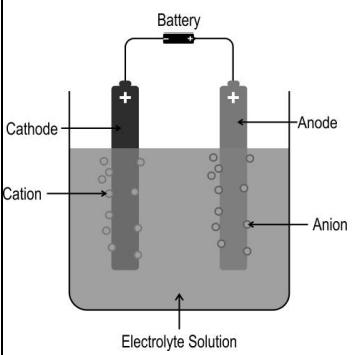Based on this, Which of the following statements is/are correct?

(i) The solution remains electrically neutral during electrolysis.

(ii) Electrons flow from the current source towards the solution at one electrode, and an

equal number of electrons flow away from the solution at the other electrode.

(iii) The number of positive ions moving towards one electrode is always equal to the

number of negative ions moving towards the other electrode.

1. i only
2. i and ii only
3. ii and iii only
4. all- i, ii, and iii

Q.6 For a certain reaction X, rate = 0.7ZABe-EA/RT.

It is seen that for another reaction, Y, rate = ZABe-EA/RT.

Based on the above, what can be said about reactions X and Y?

1. Both reactions involve complex molecules.
2. Both reactions involve simple molecules or atomic species.
3. Reaction X involves simple molecules or atomic species, while reaction Y involves complex molecules.
4. Reaction X involves complex molecules, while reaction Y involves simple molecules or atomic species.

Q.7 A metal ion Mn+ forms a complex ion of formula [ML2](n−4)+ where L represents a bidentate ligand.

Which of the following could be the charge on the ligand L?

1. -2
2. -1
3. 0
4. +2

Q.8 The image below shows different benzene derivates that give mononitration product at ortho, meta and para positions along with the rate of nitration relative to benzene.

Which of the following row shows at least one INCORRECT description of the Reaction?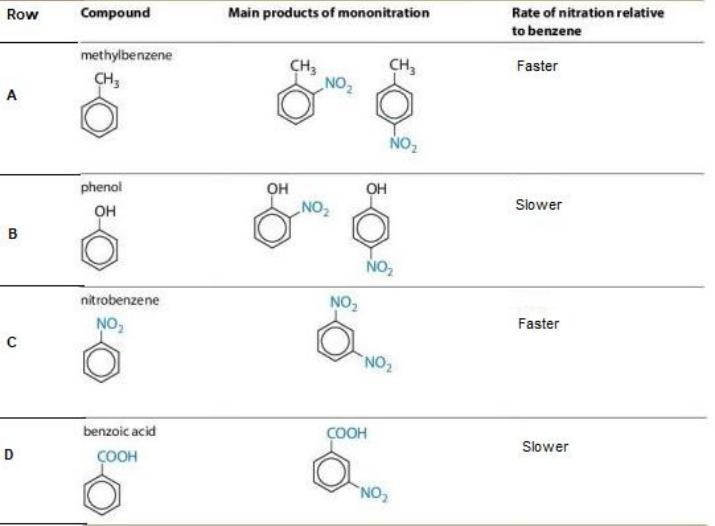1. only B
2. only C
3. only B and C
4. only C and D

Q.9 The rate constants of a reaction at 400 K and 600 K are 5 x 10-3

S–1 and 8 x 10-3s–1 respectively.

What extra piece of information is needed to calculate the value of A (frequency factor)?

(According to the Arrhenius equation, the rate constant is given by, k = Ae-Ea/RT.)

1. the order of the reaction
2. the activation energy of the reaction
3. the initial concentration of the reactants
4. [No extra information is needed. A can be calculated with the information available]

Q.10 The compound [Co(NH3)5Cl]SO4 is isomeric with the compound [Co(NH3)5SO4]Cl.

Which of the following rows correctly represents the oxidation state of cobalt in these

compounds?

Which of the following rows correctly represents the oxidation state of cobalt in these

compounds?

 Rows [Co(NH3)5Cl]SO4 [Co(NH3)5SO4]Cl A +2 +3 B +3 +2 C +2 +1 D +3 +3
1. A
2. B
3. C
4. D

Q.11 The image below shows an experimental setup to prepare an organic product X.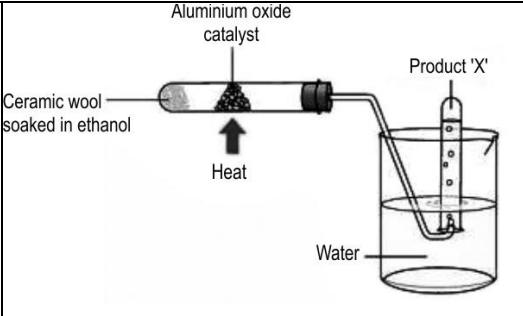Which of the following could ‘X’ be?

1. ethane
2. ethene
3. ethanoic acid
4. diethyl ether

Q.12 During protein synthesis in cells, amino acids condense (in the presence of enzymes) through the formation of the amide link (–CONH–), or peptide bond, to form a

polypeptide chain, which then folds to form a biologically active protein.

The equation below shows the formation of a dipeptide, Ala-Gly, formed by

condensation of the two amino acids, alanine and glycine in a test tube.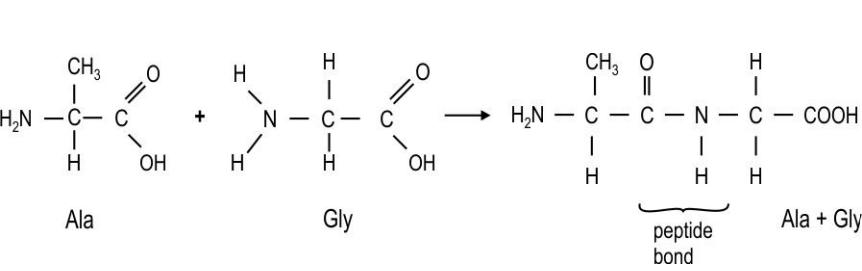Which of the following statements is/are true for the above reaction?

(i) A dipeptide Gly-Ala is equally likely to be formed by condensation of alanine and

glycine.

(ii) Water is eliminated in the above condensation reaction.

(iii) Oxygen and hydrogen is released as gases in the above condensation reaction.

1. i only
2. i and ii only
3. ii and iii only
4. all- i, ii, and iii

Q13 Zirconium (Zr, Atomic number 40) and Hafnium (Hf, Atomic number 72) are transition series metals of group 4. They are found together in nature and are difficult to separate from each other.

Which of the following is the reason for the above?

1. The almost identical radii of the atoms.
2. The elements belong to the same group.
3. The elements belong to adjacent periods.
4. The presence of the same number of unpaired electrons in both elements.

Q.14 Which of the following would be among the products of the reactions between ammonia

reacts with bromoethane?

(i) CH3CH2NH2

(ii) (CH3CH2)2NH

(iii) (CH3CH2)3N

(iv) (CH3CH2)4N+Br

1. only i
2. only i and ii
3. only i, ii, and iii
4. all- i, ii, iii and iv

Q.15 Given below are two statements labelled as Assertion (A) and Reason (R).

Assertion (A): Dimethyl amine has a higher boiling point than trimethyl amine.

Reason (R): The molecular mass of trimethyl amine is relatively higher than that of

dimethyl amine.

Select the most appropriate answer from the options given below:

1. Both A and R are true and R is the correct explanation of A.
2. Both A and R are true but R is not the correct explanation of A.
3. A is true but R is false.
4. A is false but R is true.

Q.16 Given below are two statements labelled Assertion (A) and Reason (R).

Assertion (A): A silver mirror can be created at the wall of a test tube using ethanal.

Reason (R): Ethanal can react with Fehling’s solution

Select the most appropriate answer from the options given below:

1. Both A and R are true and R is the correct explanation of A.
2. Both A and R are true but R is not the correct explanation of A.
3. A is true but R is false.
4. A is false but R is true

Q.17 Given below are two statements labelled as Assertion (A) and Reason (R).

Assertion (A): At a constant temperature, the dissociation constant of chloroethanoic acid will be higher than that of propanoic acid.

Reason (R): Higher the number of carbon atoms in a compound, the lower will be

the dissociation constant.

Select the most appropriate answer from the options given below:

1. Both A and R are true and R is the correct explanation of A.
2. Both A and R are true but R is not the correct explanation of A.
3. A is true but R is false.
4. A is false but R is true.

Q.18 Given below are two statements labelled as Assertion (A) and Reason (R).

Assertion (A): At room temperature, propan-2-ol and 2-methylpropan-2-ol, when heated

with acidified potassium dichromate, slowly turns the colour of orange dichromate to green.

Reason (R): Secondary and tertiary alcohols are readily oxidised to aldehydes which gets oxidised to acids.

Select the most appropriate answer from the options given below:

1. Both A and R are true and R is the correct explanation of A.
2. Both A and R are true but R is not the correct explanation of A.
3. A is true but R is false.
4. Both A and R are false

You can read and download the complete practice paper for CBSE class 12 Chemistry exams 2023 below.

Also Check: CBSE Class 12th Chemistry Notes

CBSE Class 12 Chemistry Sample Paper 2022-23

CBSE Class 12 Chemistry Deleted Syllabus 2022-23

CBSE Chemistry Previous Year Question Paper Class 12

NCERT Laboratory Manual for CBSE Class 12 Chemistry: Practicals & Projects

Other Important resources for CBSE 12th Exams 2023.